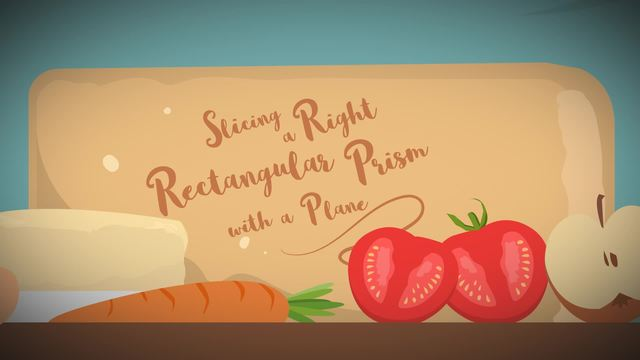# Slicing a Right Rectangular Prism with a PlaneRate this video

Ø 5.0 / 2 ratings

The authorNicholas V.

## Information about the videoSlicing a Right Rectangular Prism with a Plane

After this lesson, you will be able to describe the shapes created by slicing a right rectangular prism.

The lesson begins by teaching you that slicing creates a plane section. It leads you to learn that slices perpendicular to the base and parallel to a face create rectangles congruent to that face. It concludes with an exploration of other ways of slicing the prism.

Learn about slicing right rectangular prisms by helping Tom and Eddy decide how to divide their room!

This video includes key concepts, notation, and vocabulary such as a right rectangular prism (a figure composed of two congruent rectangular bases and 4 rectangular lateral faces that are perpendicular to the bases); plane (a flat region extending infinitely in two dimensions); and plane-section (the 2-dimensional shape formed by the intersection of a plane and a prism or other polyhedron).

Before watching this video, you should already be familiar with right rectangular prisms, rectangles, right angles, area, volume, and the idea of congruence.

After watching this video, you will be prepared to learn about the plane-sections formed when right rectangular prisms are sliced at angles and when pyramids are sliced.

Common Core Standard(s) in focus: 7.G.A.3 A video intended for math students in the 7th grade Recommended for students who are 12 - 13 years old

### TranscriptSlicing a Right Rectangular Prism with a Plane

Tom and his brother Eddy aren't so happy about their shared bedroom. Eddy leaves his gym clothes everywhere and Tom's toad acts like it owns the place. The only thing they don't fight about is lunch. Maybe making some sandwiches will help them separate their room and get some peace. In order to do so, Tom and Eddy will need to learn how to slice a right rectangular prism, with a plane. What does it mean to slice something in real life? When we slice a loaf of bread, a tomato, an apple, or salami we make a flat cut through the object, going straight through without curving. The slice divides the 3-D shape into 2 pieces and also leaves us a two-dimensional shape on the outside. Let's look at the loaf of bread. What shape is it? It's a rectangular prism, since it has two congruent bases... both of which are rectangles. In fact, since these angles are right angles, this is a right rectangular prism. That means, that the lateral faces will also be rectangles. There are lots of ways to slice a loaf of bread, but usually we slice it like this. A slice can be represented by a plane intersecting the 3-D shape. Remember, a plane is a flat, 2-dimensional shape that extends out infinitely in all directions. In this case, the intersecting plane is perpendicular to the top and bottom of the prism, and parallel to the ends. What 2-dimensional shape will be created by the slice? It's a rectangle. And this rectangle is congruent to the faces it was parallel to: the ends of the prism. We call the 2-d shape created by the intersection of a plane and a 3-d figure, a plane section. Slicing right rectangular prisms like this will always give us a plane section that is a rectangle congruent to the ends. That's the greatest thing since sliced bread! Now let's look at another right rectangular prism and see what happens if we rotate our knife and then slice. Now the plane is perpendicular to the base but not parallel to the ends. What's the shape of the resulting plane section? Let's look at it from another angle. Ah. Now we can see plain as day: it's another rectangle! It has the same height as the prism but the width of the rectangle is longer than the width of the end. It's the same type of shape, but it's neither congruent nor similar. Finally, let's look at what happens if the intersecting plane is parallel to the base of the right rectangular prism. What shape do you think the resulting plane section will be? It's another rectangle and this time it's congruent to the base. While the boys finish up lunch and decide what to do about their room, let's review. Intersecting a 3-d figure with a plane is known as slicing. Slicing results in a 2-d figure, that we call a plane section. If we slice parallel to two of the lateral faces of a right rectangular prism the plane section is a rectangle that is congruent to those faces. If we slice parallel to the base the plane section is a rectangle that is congruent to the base. And if we slice perpendicular to the base, but not parallel to any of the lateral faces we also get a rectangle, but it isn't congruent to any of the faces. The boys have taken their knowledge of slicing and sliced the room in half, using a rectangular shaped curtain. Wow, it's actually quiet! But it's also kinda boring. No matter how you slice it, Tom and Eddy are better together!